# Natural Draught and Chimney

Draught is the pressure difference which causes a flow of air or gases from one point to another point in the boiler system. Draught is required in a boiler system mainly due to two reasons.

1. To supply sufficient air for completing the combustion.
2. To remove flue gases from the system after combustion and the heat exchange.

There are two types of draught applied to the boiler system.

1. The natural draught
2. The forced draught

We will discuss here in this article about natural draught. The natural draught is always preferred since it does not need any running cost although it has a big initial cost. Natural draught allows natural circulation of air through the boiler system. The natural draught mainly depends upon the height of the chimney.

We try to calculate the required height of the chimney for a required natural draught for a boiler system. For that, we have to go through two basic equations of gaseous pressure. The equations are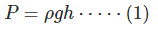Where, “P” is the pressure of air or gas, “ρ” is the density of the air or gas, “g” is the gravitational constant, and “h” is the height of the head.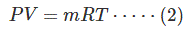Here “V” is the volume of the air or gas, “m” is the mass of the gas or air, “T” is the temperature measured in kelvin scale and “R” is the gas constant.
Equation (2) can be rewritten as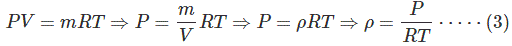During combustion process in the furnace, mainly carbon reacts with oxygen (O2) of the air and forms carbon dioxide (CO2). The volume of the solid carbon compared to the required air for the reaction is negligible. Because of that, we can consider that the volume of air required for combustion is exactly equal to the volume of the flue gases created after combustion if we assume temperature before and after combustion are same. But this is not the actual case. The air entries in the combustion chamber will gain extra volume after combustion due to combustion temperature. The gained volume of the air will be equivalent to the volume of the flue gases created after combustion.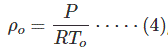Let us assume, ρo is the density of the air at 0oC or 273 K, and say it is To
Here, P is the pressure of air at 0oC or 273 K, that is at To K.
If we keep, the pressure P as constant, the relation between density and temperature of the air or gases can be written as,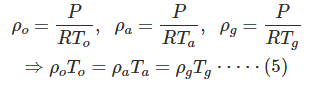Where, ρa and ρg is the density of the air at temperature Ta and Tg K respectively.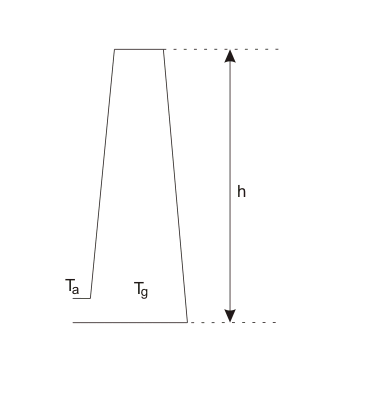From, equation (1) and (5) we can write the expression of pressure at point “a” outside the chimney, as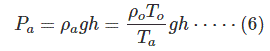The volume of the air at the temperature Tg would be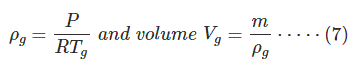Let us assume, m kg of air is required to burn 1 kg of carbon then the density of the flue gas would be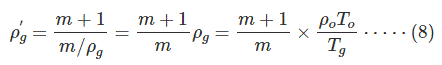The pressure of the flue gas inside the cheimny from equation (1) and (8), would be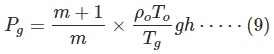The pressure difference between outside and inside of the chimney from equation 96) and (9) would be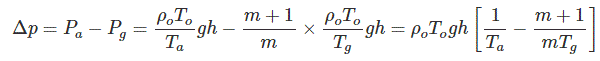Here, “h” is the minimum height of the chimney to be constructed for the draught ΔP. The flue gas will flow upwards through the chimney due to this pressure difference. So, by calculating this pressure difference one can easily calculate the approximate height of the chimney to be constructed. The pressure difference can be represented as a formula for calculating the height of the chimney for a natural draught.

Want To Learn Faster? 🎓
Get electrical articles delivered to your inbox every week.
No credit card required—it’s 100% free.